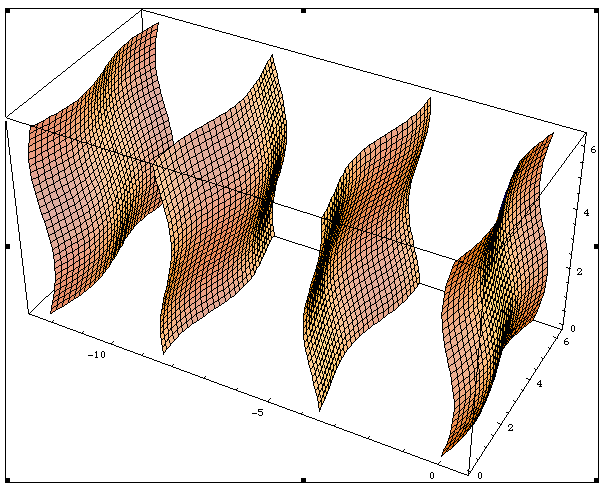# Superspace

### From Online Dictionary of Crystallography

Superespace, hyperespace (Fr). Superraum (Ge). Superspazio (It). 超空間 (Ja). Superespacio (Sp).

## Definition

Superspace is a Euclidean vector space with a preferred (real) subspace VE that has the dimension of the physical space, usually three, but two for surfaces, and one for line structures.

## Applications

Superspace is used to describe quasi-periodic structures (see aperiodic crystal). We denote the dimension of the physical space by m. Then a function ρ(r) is quasi-periodic if its Fourier transform is given by

$\rho( r)~=~\sum_{ k \in M^*} \hat{\rho}( k \exp (2\pi i k. r ),$

where the Fourier module M* is the set of vectors (Z-module) of the form

$k~=~\sum_{i=1}^n h_i a_i^* ~~~(h_i~{\rm integers}),$

for a basis ai* with the property that $\sum_i h_i a_i^*=0$ implies hi=0 (all i) if the indices hi are integers. The number n of basis vectors is the rank of the Fourier module.

The vectors rs have two components: r and rI. The relation is written as

$r_s~=~( r,~ r_I).$

In the superspace there is a reciprocal basis asi* such that ai* is the projection of asi* on the subspace VE. The reciprocal lattice Σ in superspace then is projected on the Fourier module M*. The function ρ(r) then can be embedded as

$\rho_s(r_s)~=~\sum_{k_s\in\Sigma^*} \hat{\rho}(\pi k_s= k) \exp (2\pi i k_s.r_s).$

The function ρ(r) is the restriction of ρs(rs) to the subspace

$\rho( r)~=~\rho_s( r,0).$

Because the Fourier components in superspace belong to reciprocal vectors of Σ*, the function ρs(rs) is lattice periodic. Its direct lattice Σ is the dual of Σ* and its basis vectors are ais satisfying asi.asj* = δij. Therefore, the symmetry group of ρs(rs) is a space group in the n-dimensional superspace, where the dimension n is equal to the rank of the Fourier module. In the superspace the usual crystallographic notions and techniques may be applied. For point atoms, the function ρs(rs) is concentrated on surfaces of co-dimension equal to the dimension of the physical space (see figure).An example of the embedding of a one-dimensional modulated chain with two modulation wave vectors. It consists of an array of two-dimensional surfaces, with three-dimensional lattice periodicity. The intersection of the surfaces with the physical space (a line) gives the positions of the modulated structure.